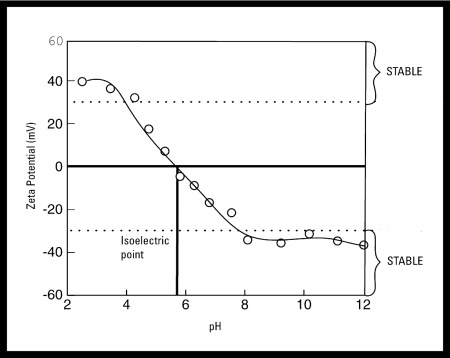Colloidal Silver | Silver Colloids# The Influence of Zeta Potential and pH

•  The most important factor that affects zeta potential is pH
• A zeta potential value quoted without a definition of it’s environment (pH, ionic strength, concentration of any additives) is a meaningless number

## Why Does Zeta Potential Change?

Imagine a negative zeta potential in suspension

• If more alkali is added to this suspension then the particles tend to acquire more negative charge
• If acid is added to this suspension then a point will be reached where the charge will be neutralized
• Further addition of acid will cause a build up of positive charge
• In general, a zeta potential versus pH curve will be positive at low pH and lower or negative at high pH
• There may be a point where the curve passes through zero zeta potential
• This point is called the isoelectric point and is very important from a practical consideration
• It is normally the point where the colloidal system is least stableWhy Does Zeta Potential Change – Zeta Potential and Ph Chart

In the above example it can be seen that if the dispersion pH is below 4 or above 8 there is sufficient charge to confer stability. However if the pH of the system is between 4 and 8 the dispersion may be unstable. This is most likely to be the case at around pH 6 (the isoelectric point)